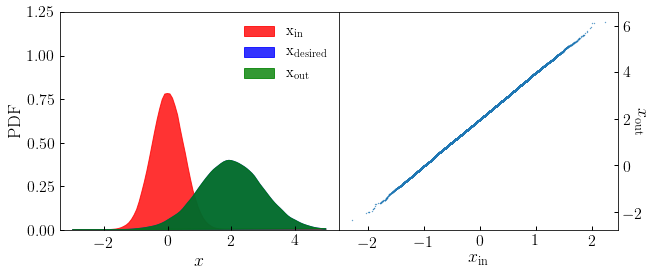# bijective_distribution_matching¶

halotools.utils.bijective_distribution_matching(x_in, x_desired)[source] [edit on github]

Replace the values in x_in with x_desired, preserving the rank-order of x_in

Parameters: x_in : ndarray Numpy array of shape (npts, ) x_desired : ndarray Numpy array of shape (npts, ) x_out : ndarray Numpy array of shape (npts, )

Examples

>>> npts = int(1e5)
>>> x_in = np.random.normal(loc=0, scale=0.5, size=npts)
>>> x_desired = np.random.normal(loc=2, scale=1, size=npts)
>>> x_out = bijective_distribution_matching(x_in, x_desired)


In the figure below, the left hand panel shows that the output distribution is in exact agreement with the desired distribution. The right hand panel shows that the rank-order of the input distribution is preserved.